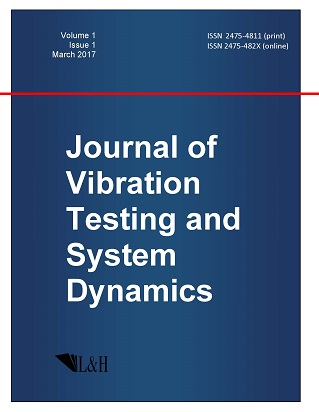ISSN: 2475-4811 (print)
ISSN: 2475-482X (online)
Journal of Vibration Testing and System Dynamics

C. Steve Suh (editor), Pawel Olejnik (editor),

Xianguo Tuo (editor)

Pawel Olejnik (editor)

Lodz University of Technology, Poland

Email: pawel.olejnik@p.lodz.pl

C. Steve Suh (editor)

Texas A&M University, USA

Email: ssuh@tamu.edu

Xiangguo Tuo (editor)

Sichuan University of Science and Engineering, China

Email: tuoxianguo@suse.edu.cn

Analytical and Numerical Methods in Differential Equations for the 100th Birthday Anniversary of Nikolai N. Yanenko

Journal of Vibration Testing and System Dynamics 7(1) (2023) 1--3 | DOI:10.5890/JVTSD.2023.03.001

S. V. Meleshko$^1$, S. Moyo$^2$, E. Schulz$^1$, J. Tanthanuch$^1$

$^1$ School of Mathematics, Institute of Science, Suranaree University of Technology, Nakhon Ratchasima, 30000, Thailand

$^2$ Office of the DVC Research, Innovation and Engagement & Institute for Systems Science, Durban University of Technology, Durban 4000, South Africa

Abstract

This special topic section collects recent developments on methods in differential equations dedicated to Nikolai Yanenko's 100th birthday anniversary. The papers were presented at the International Conference `Analytical and Numerical Methods in Differential Equations (Yanenko 100)' which was held during 23-27 August 2021. %\includegraphics[width=0.2\textwidth]{Yanenko2.jpg} This conference was a celebration of Yanenko's foundational contributions on analytical and numerical methods in differential equations. Its topics ranged from physical experiment and mathematical modelling of real problems all the way to abstract mathematical objects. The 6 papers published herein were selected from the 76 presentations to share these advanced developments in differential equations with the community of Journal of Vibration Testing and System Dynamics.

References

1.   Campoamor-Stursberg, R. (2023), Branching rules and subduced representations applied to Lie point symmetries of differential equations, Journal of Vibration Testing and System Dynamics, 7, 5-14.
2.   Kovtunenko, V.A. (2023), Mixed variational problem for a generalized Darcy–Forchheimer model driven by hydraulic fracture, Journal of Vibration Testing and System Dynamics, 7, 15-21.
3.   Mitsopoulos, A. and Tsamparlis, M. (2023), Higher order first integrals of autonomous dynamical systems in terms of geometric symmetries, Journal of Vibration Testing and System Dynamics, 7, 23-30.
4.   Rogalev, A.N. (2023), Symbolic methods for estimating the sets of solutions of ordinary differential equations with perturbations on a finite time interval, Journal of Vibration Testing and System Dynamics, 7, 31-37.
5.   Vedenyapin, V.V., Fimin, N.N., Russkov, A.A., Voronina, M.Yu. (2023), On the derivation of the equations of gravitation and electrodynamics from the generalized least action principle and the nonrelativistic models of the universe, Journal of Vibration Testing and System Dynamics, 7, 39-47.
6.   Siriwat, P. and Meleshko, S.V. (2023), Methods for constructing reciprocal transformations, Journal of Vibration Testing and System Dynamics, 7, 49-58.